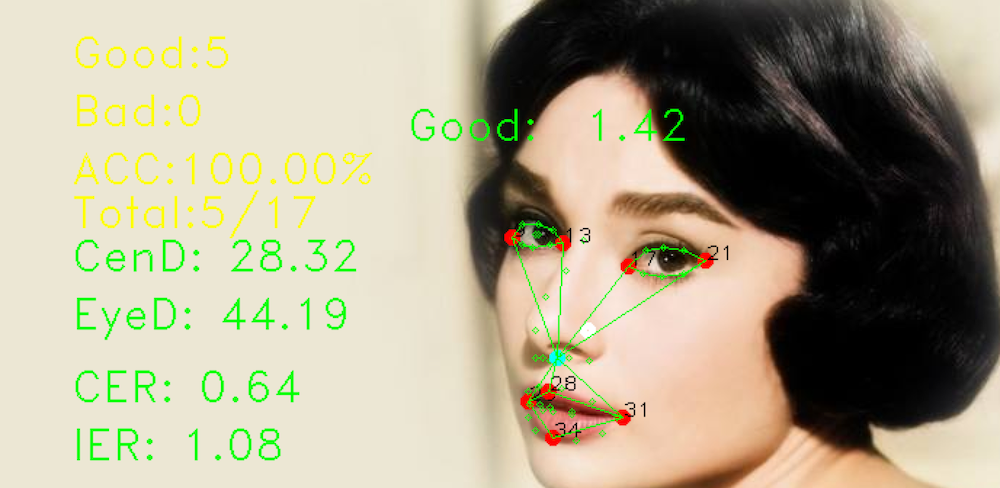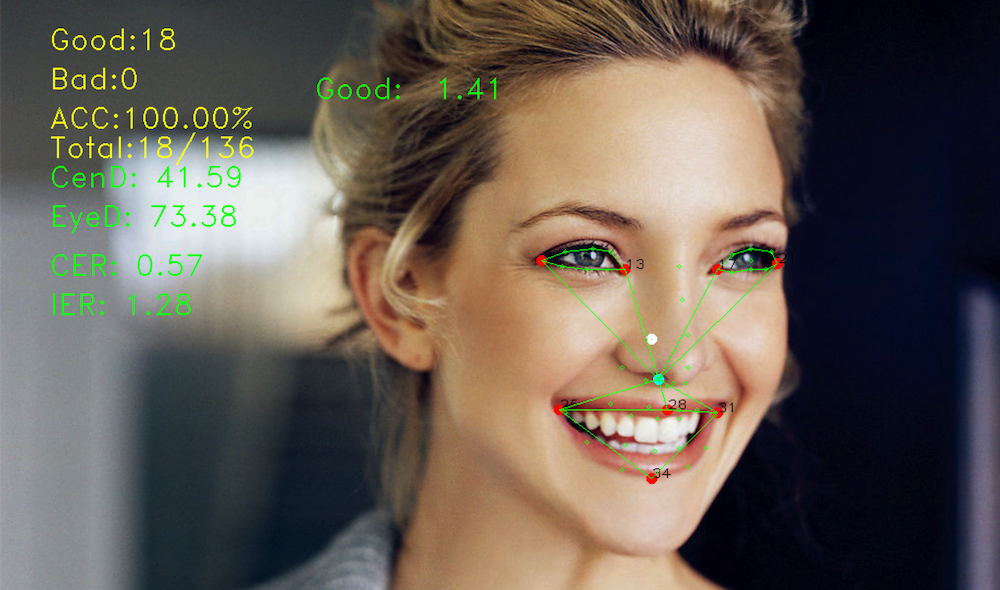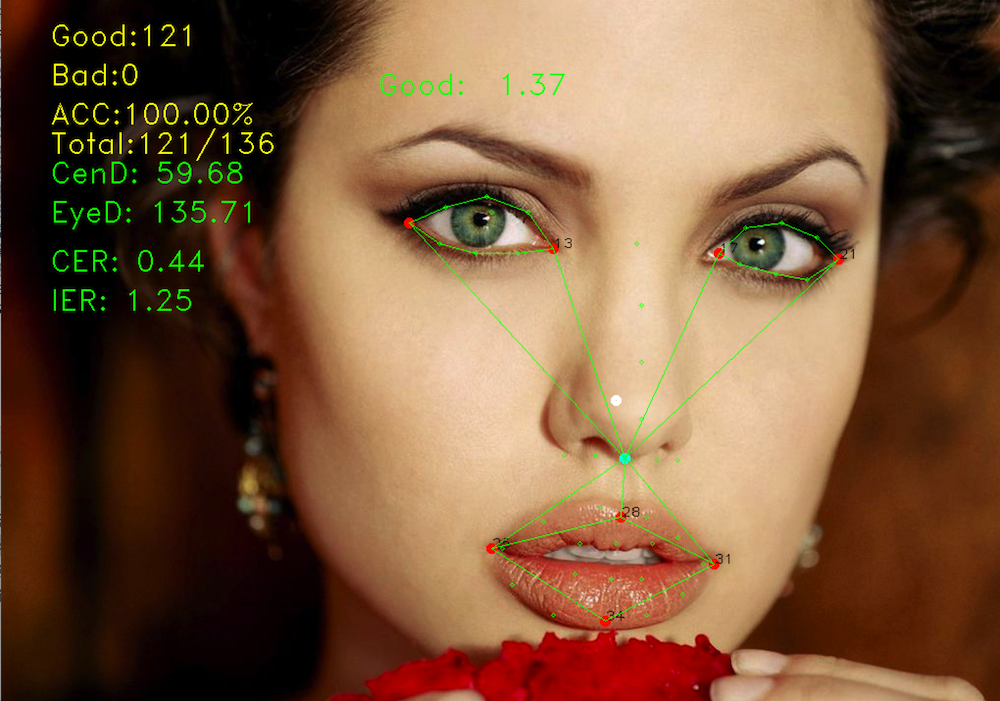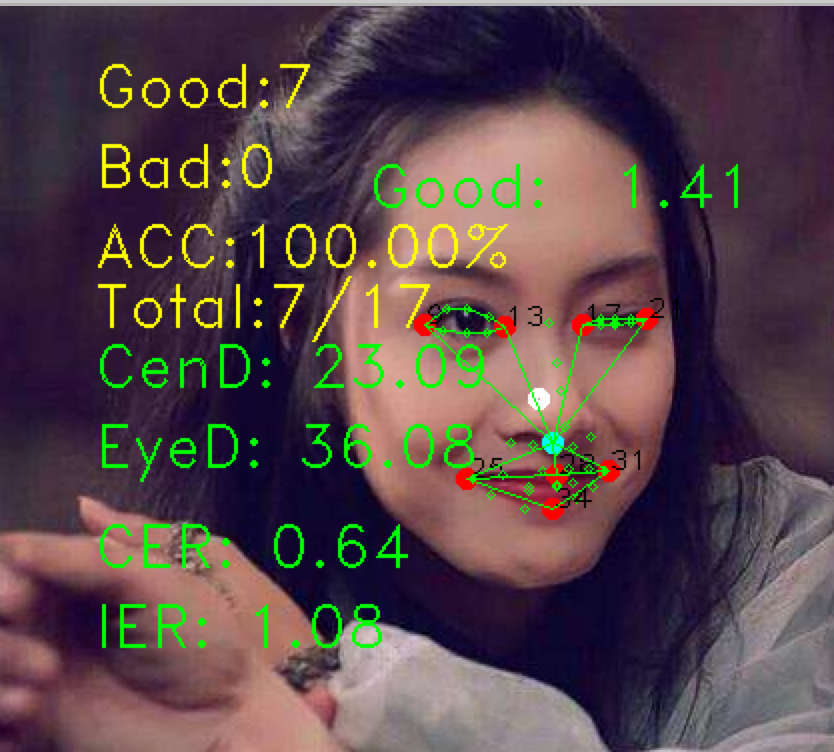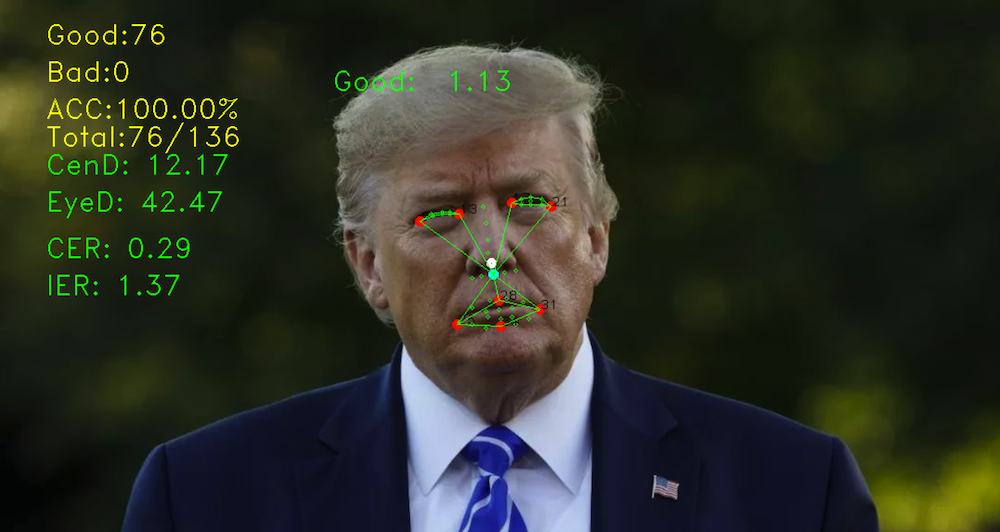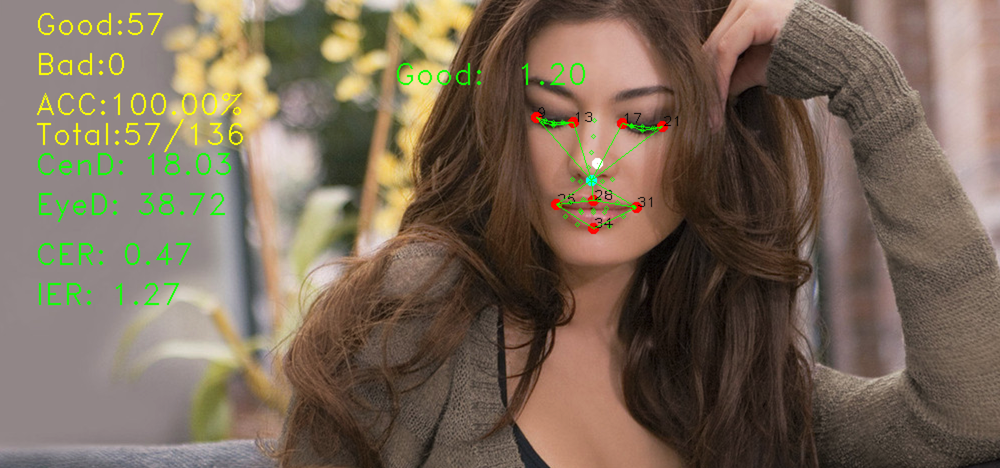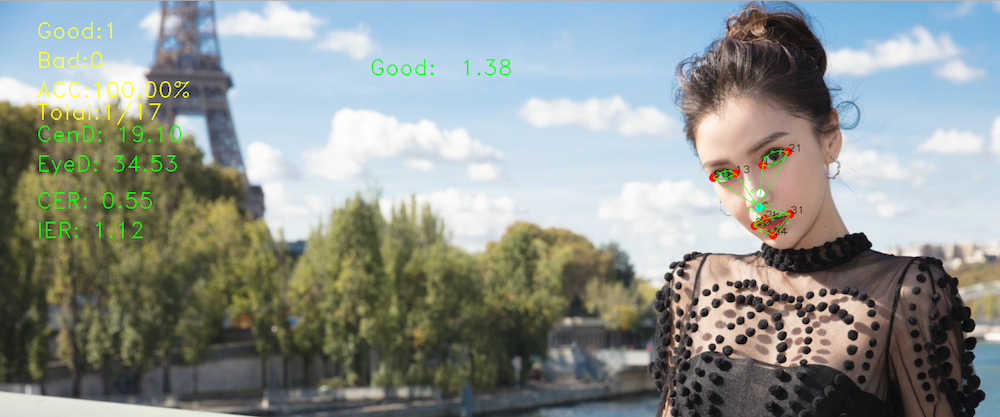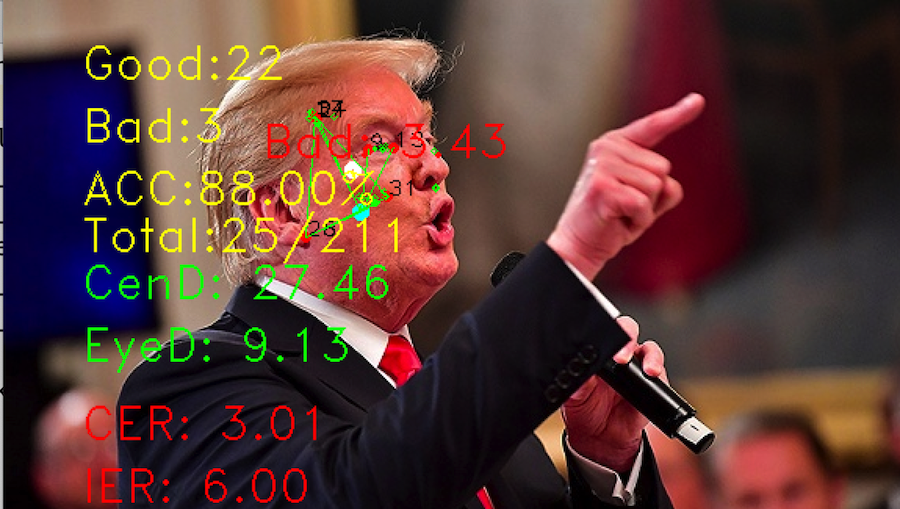2019/10/08 - 次.

# 人脸特征点置信度算法

## 一、 FCC(Facial Landmark Confidence Coefficient)

### 1. 人中中点与眼睛的系数

$NC = mean(n_i)i\in[1, 5]$ $E_1$ = $\sqrt{NC^2+[mean(L_i)i\in[1,2]]^2}$ $E_2$ = $\sqrt{NC^2+[mean(R_i)i\in[1,2]]^2}$ $E_3$ = $\sqrt{NC^2+[mean(L_i)i\in[1,8]]^2}$ $E_4$ = $\sqrt{NC^2+[mean(R_i)i\in[1,8]]^2}$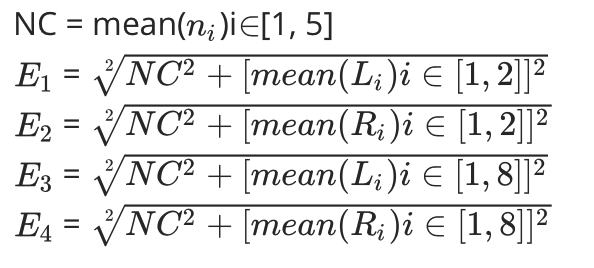• NC为人中5点的均值坐标，或者称为人中的中点坐标
• $E_i$是NC到左右眼的各均值点的距离

$Eye Coefficient = \frac{1}{4}\displaystyle \sum^{4}_{i=1}E_i$Eye Coefficient表示人中中点与眼睛的系数，该系数主要表示为人中中点到左右眼各均值点的距离平均值，其中$E_1$E1是NC到$L_1$L1和$L_2$L2的均值点距离，同理$E_3$E3是NC到$L_1$L1-$L_8$L8, 8点均值的距离。

### 2. 人中中点与嘴角的系数

$Mouth Coefficient = \frac{1}{4}\displaystyle \sum^{4}_{i=1} \sqrt{NC^2+m^2_i}$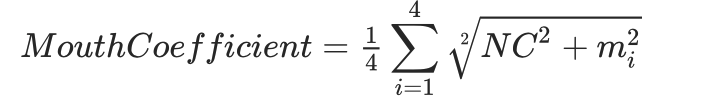• $m_i$m_i 嘴角的4点坐标
• Mouth Coefficient 是NC到嘴角4点的距离均值

### 3. 眼睛与嘴角系数的比

• EMR 可以用来表示以NC为比例点，人脸上半部分与下半部分的比例系数，该系数可以用来粗略定位人脸整体的关系，特征点是否存在大的偏差。

### 5. 左右眼眼宽系数

$min(\sqrt{L^2_1+L^2_2}, \sqrt{R^2_1+R^2_2})$ $max(\sqrt{L^2_1+L^2_2}, \sqrt{R^2_1+R^2_2})$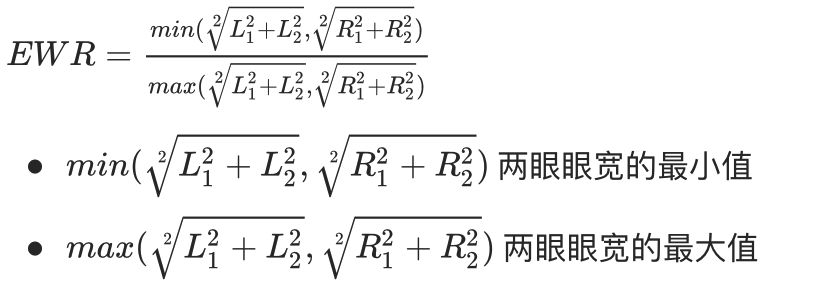### 6. 面部置信度系数

$FCC = \frac{1}{3}(EMR + EDR + EWR)=\frac{1}{3}(\frac\sum^{4}_{i=1}E_i}\sum^{4}_{i=1} \sqrt{NC^2+m^2_i}} + \sqrt{\frac{L^2_1+R^2_1}{L^2_2+R^2_2}} + \frac{min(\sqrt{L^2_1+L^2_2}, \sqrt{R^2_1+R^2_2})}{max(\sqrt{L^2_1+L^2_2}, \sqrt{R^2_1+R^2_2})}$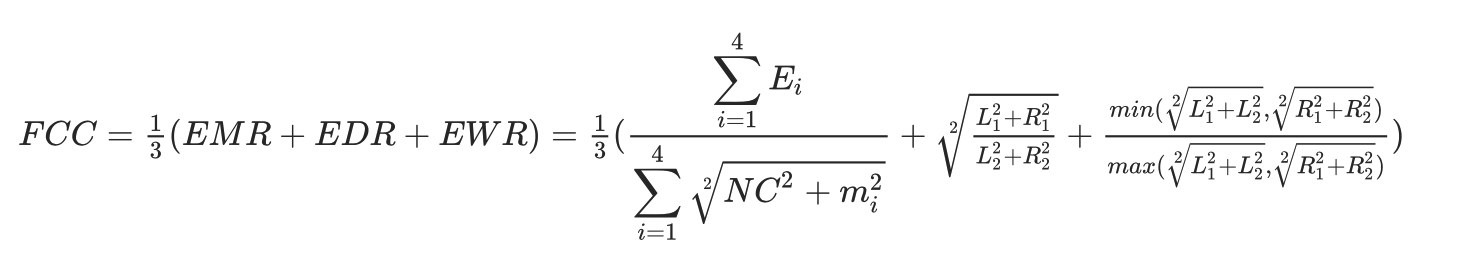• FCC 面部置信度系数， 0.85 < FCC <= 1.9

## 二、辅助系数CER

CER可以很方便的检测出面部四角区域的混乱程度，当值超出范围值时，眼睛与嘴角会出现较大的扭曲。 $CER = \frac{2\sqrt{NC^2 + [mean(L_i, R_i, M_j)i\in[1,2],j\in[1,4]]^2}}{\sqrt{L^2_1+L^2_2} + \sqrt{R^2_1+R^2_1}}$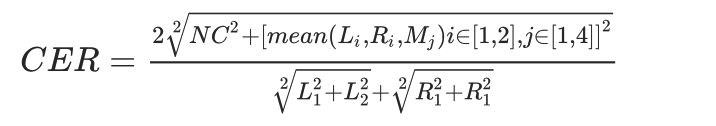• CER(Face Center Eye Ratio)是用于恒量左眼、右眼和嘴角所连多边形混乱程度的系数
• 1.2 < CER <= 0

## 三、辅助系数IER

IER可以近一步规范眼睛区域的特征点，例如常见的大小眼、左右眼重叠、眼距过大或过小等情况 $IER = \frac{2\sqrt{L^2_2+R^2_1}}{\sqrt{L^2_1 + L^2_2} + \sqrt{R^2_1 + R^2_2}}$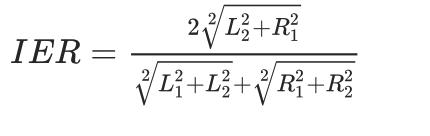• IER(Inside Eye Ratio)
• 0.8 <= IER <= 3.5

## 四、实际检测效果